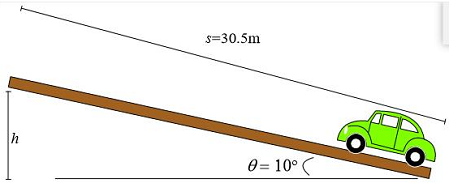# You are driving your car uphill along a straight road. Suddenly, you see a car run a red light...

## Question:

You are driving your car uphill along a straight road. Suddenly, you see a car run a red light and enter the intersection just ahead of you. You slam on your brakes and skid in a straight line to a stop, leaving skid marks 100 feet (30.5 m) long. A policeman observes the whole incident and gives a ticket to the other car for running the red light. He also gives you a ticket for exceeding the speed limit of 30 mph (13.4 m/s). You are pretty angry about getting this ticket. When you get home, you read your physics book and estimate that the coefficient of kinetic friction between your tires and the road is 0.60, and the coefficient of static friction is 0.80. You estimate that the hill made an angle of about 10 degrees with respect to the horizontal. You look in your owner's manual and find that your car weighs 2,050 lbs (932 kg). Use conservation of energy to decide whether or not it is worth fighting the ticket in court?## Friction:

The frictional force acts such that it reduces the velocity of an object.

The direction of the frictional force and the initial velocity of the object are opposite.

Given:

• The mass of the car is {eq}m = 932\ \text{kg} {/eq}.
• The inclination angle is {eq}\theta = 10^\circ {/eq}.
• The stopping distance is {eq}s = 30.5\ \text{m} {/eq}.
• The coefficient of kinetic friction between the tires and the road is {eq}\mu_k = 0.60 {/eq}.
• Let the initial speed of the car be {eq}v {/eq}.

The initial kinetic energy of the car is equal to the sum of the work done by the frictional force on the car and the final gravitational potential energy.

\begin{align} 0.5\ m\ v^2 &= \mu_k\ m\ g \ s\ \cos\theta + m\ g \ h\\ 0.5\ v^2 & = \mu_k\ g \ s\ \cos\theta + g \ h\\ 0.5\ v^2 & = \mu_k\ g \ s\ \cos\theta + g \ s\ \sin\theta\\ 0.5\ v^2 & = (0.60)\ (9.81\ \text{m/s}^2)\ (30.5\ \text{m})\cos\ 10^\circ + (9.81\ \text{m/s}^2)\ (30.5\ \text{m})\ \sin\ 10^\circ\\ 0.5\ v^2 & = 176.8\ \rm{m^2/s^2} + 51.96\ \rm {m^2/s^2}\\ \implies v &= 21.4\ \text{m/s}\\ \end{align}.

The car was speeding so it is not worth fighting the ticket in court.Characteristics & Effects of Friction

from

Chapter 5 / Lesson 10
16K

Friction is the force that resists motion. An object's weight and surface material can affect the amount of friction present. Learn how to increase and decrease friction as well as the difference between static and kinetic friction.